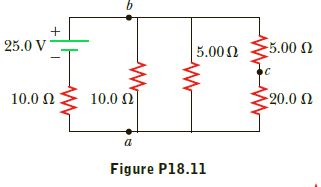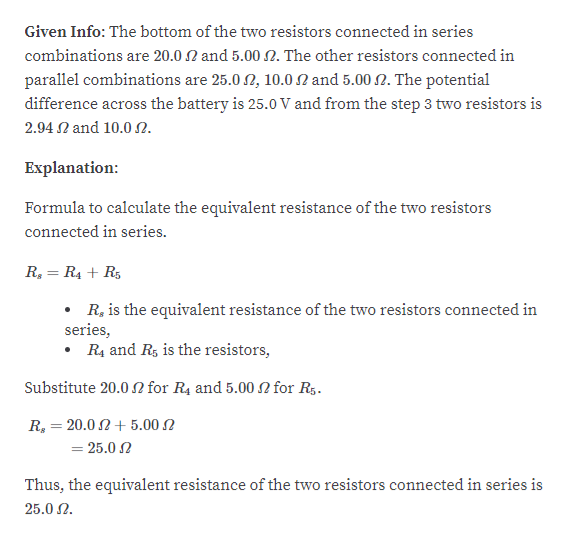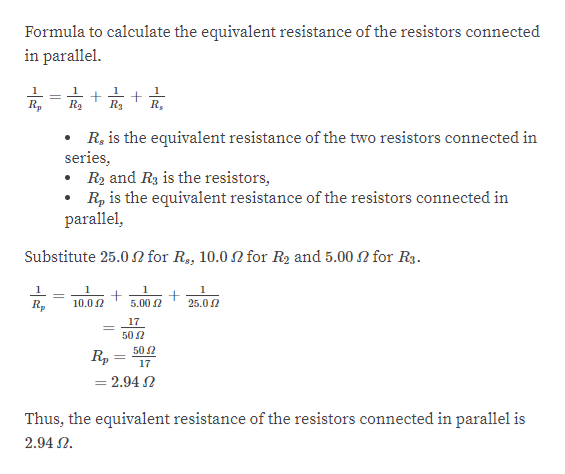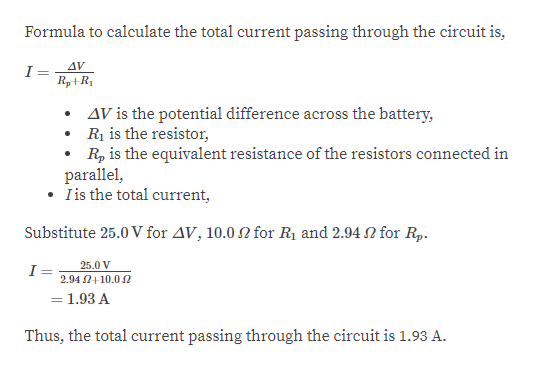Question
2 views

Consider the circuit shown in Figure P18.11. Find (a) the
potential difference between points a and b and (b) the current
in the 20.0 - Ω resistor.help_outlineImage Transcriptionclose5.00 2 25.0 V 5.00N 10.0 N 20.0 2 10.0 N Figure P18.11 fullscreen
check_circle

Step 1help_outlineImage TranscriptioncloseGiven Info: The bottom of the two resistors connected in series combinations are 20.0 N and 5.00 N. The other resistors connected in parallel combinations are 25.0 2, 10.0 N and 5.00 2. The potential difference across the battery is 25.0 V and from the step 3 two resistors is 2.94 N and 10.0 N. Explanation: Formula to calculate the equivalent resistance of the two resistors connected in series. R, = R4 + R5 • R, is the equivalent resistance of the two resistors connected in series, R4 and Rs is the resistors, Substitute 20.0 N for R4 and 5.00 2 for R5. R, = 20.0 2 + 5.00 N = 25.0 2 Thus, the equivalent resistance of the two resistors connected in series is 25.0 2. fullscreen
Step 2help_outlineImage TranscriptioncloseFormula to calculate the equivalent resistance of the resistors connected in parallel. %3D Rp Ry R3 R, R, is the equivalent resistance of the two resistors connected in series, • R2 and R3 is the resistors, R, is the equivalent resistance of the resistors connected in parallel, Substitute 25.0N for R, 10.0 for R2 and 5.00N for R3. 10.0 2 5.00 2 25.0 2 R, 17 50 2 50 2 R, = = 2.94 N 17 Thus, the equivalent resistance of the resistors connected in parallel is 2.94 N. fullscreen
Step 3help_outlineImage TranscriptioncloseFormula to calculate the total current passing through the circuit is, AV R,+R1 AV is the potential difference across the battery, R is the resistor, R, is the equivalent resistance of the resistors connected in parallel, • Iis the total current, Substitute 25.0 V for AV, 10.0 N for R1 and 2.94 N for Rp. 25.0 V 2.94 2+10.0 2 =1.93 A Thus, the total current passing through the circuit is 1.93 A. fullscreen

### Want to see the full answer?

See Solution

#### Want to see this answer and more?

Solutions are written by subject experts who are available 24/7. Questions are typically answered within 1 hour.*

See Solution
*Response times may vary by subject and question.
Tagged in

### Current Electricity